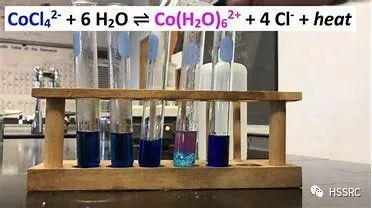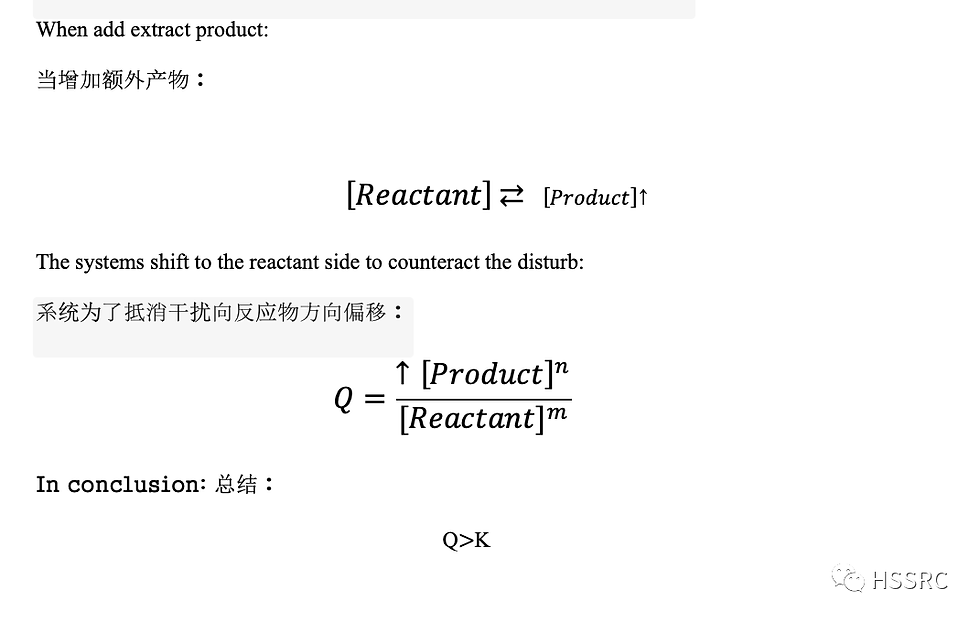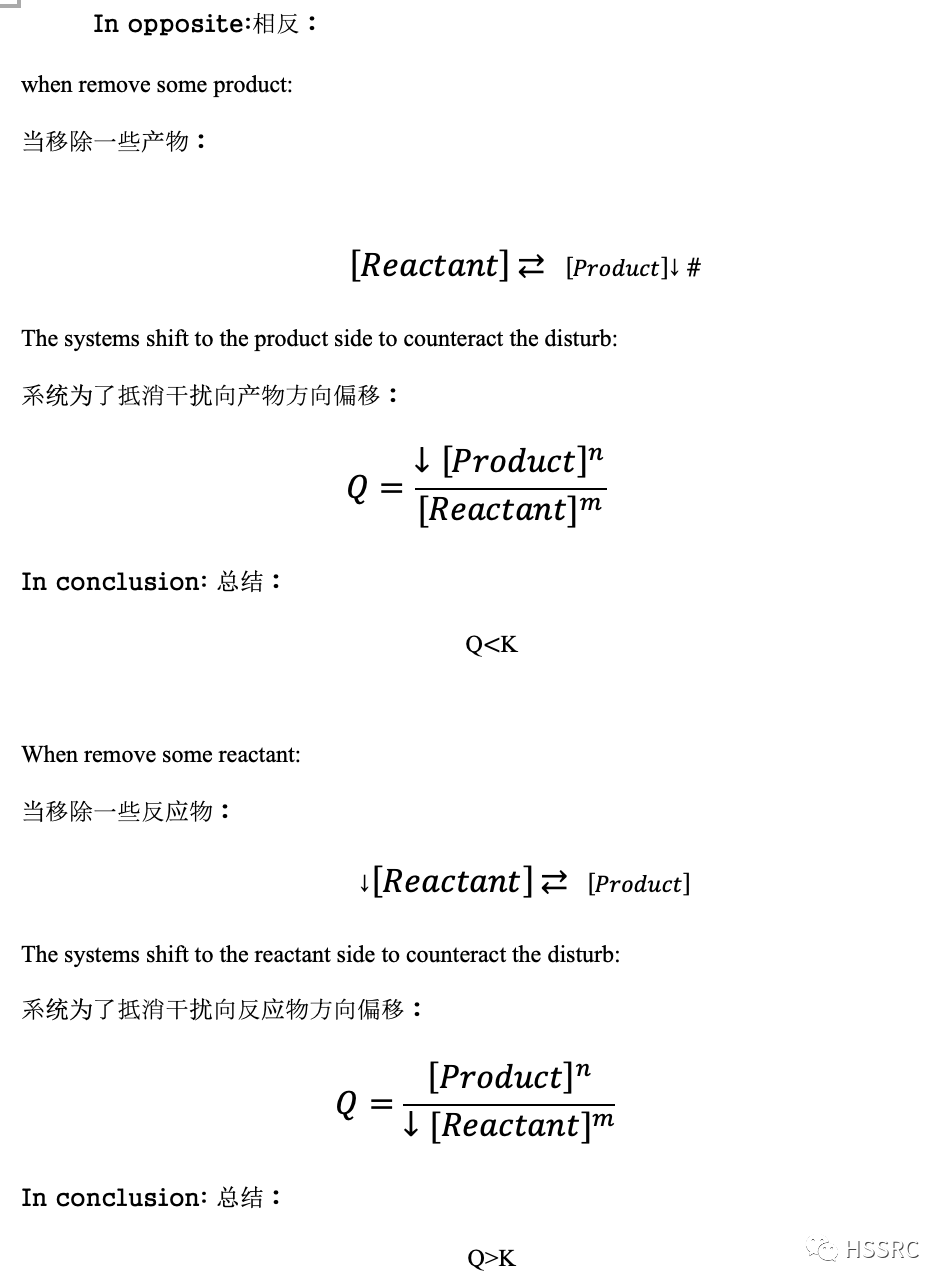top of page

# Le Chatelier’s Lab Discussion

Zixuan Huang

1

Le Chatelier's principle is an extension of equilibrium theory which explains the response of chemical systems in order to minimize the disturbance which breaks the equilibrium state. In other words, it described what the chemical systems would do to keep equilibrium constant.

2

In order to understand the Le Chatelier principle, we need to know the reason why a reversible chemical reaction would always reach the equilibrium stage at a certain point. One of the fundamental principles of chemistry explains this phenomenon. The ultimate goal of all atoms is to stay in the lowest state level, so the chemical system will always reach equilibrium at a certain point no matter how hard the system is disturbed. Because equilibrium occurs at the moment the rate of reverse reaction and rate forward reaction are the same. Which means the changing concentration of reactant and changing concentration product are the same. So a chemical system is stable and it requires the least amount of energy to maintain this condition.When equilibrium has been disturbed, the Le Chatelier principle participated. In order for a chemical system striking at equilibrium constant again, the system shifts in a direction that minimizes the disturbance. The three means to disturb the equilibrium: changing the concentration, changing the pressure or volume, and changing the temperature.

Le Chatelier’s Lab Discussion

In addition, at a specific temperature, the equilibrium constant (K) is almost unchanged for its chemical system. When the system is disturbed, the equilibrium is destroyed, K (equilibrium constant) cannot be used to describe this stage. Instead Q took its place. Because Q is a measure of the relative amounts of products and reactants present in a reaction at any given time. In meantime, at equilibrium K= Q.

According the definition of when equilibrium would occur, Scientist concluded the formula of equilibrium constant:As long as the chemical reaction reach equilibrium the final concentration of reactant or product would remain constant, therefore changing the concentration definitely are the means of disturbing the equilibrium, if an extra Product or Reactant is added, according to Le Chatelier principle, the systems will start to shifts at this point to minimize the disturbed.

Next let’s explore how systems shift. In short term the Kc formula can simplify to:Le Chatelier’s Lab DiscussionLe Chatelier’s Lab DiscussionHowever, since the system reaches equilibrium again the K will go back to its original value. Because product and reactant both increase or decrease follows either one of the product and reactant change amount. The amount they increase or decrease counteract each other.

Le Chatelier’s Lab Discussion

Changing pressure and volume is also a way to change concentration and therefore it is also a way to disturb equilibrium. We can reveal the relationship between pressure, volume and temperature from ideal gas law:

PV=nRT

P is inversely proportional to V, V inversely proportional to n, n is proportional to P.Because pressure has a direct relationship with concentration therefore we can use pressure to calculate the K also.When a pressure of a container increase will be leading the volume of the container to decrease which cause the concentration increase because M=n/v . In order to release the pressure or decrease the concentration, the system will shift in the direction that has fewer moles of gas particles.

When a pressure of a container increase will be leading the volume of the container to decrease which cause the concentration increase because . In order to release the pressure or decrease the concentration, the system will shift in the direction that has fewer moles of gas particles.1

On the reactant side, there are three moles of gas moving in the container, so they are more crowded, which leads to higher pressure, but on the product side, there are only two moles of gas moving in the container, so it will be less crowded, and the pressure will be lower. So, the reactant will quickly synthesize the product in order to release pressure. Which means the system will shift in the direction that has fewer numbers of moles of gas particles.

2

In the same way, after the pressure in the container decreases, the volume will become larger, so that there will be more space in the container. In order to fill the extra space, the system will shift in the direction that has a greater number of moles of gas particles.

To recall the equilibrium occurs when the forward reaction rate equals the reverse reaction rate, but when the temperature changes which means the average kinetic energy also changes which causes the moving speed of molecules to change. Wherefore, the break the balance of constant △[Reactant]= △[Product]. Therefore, temperature change will cause equilibrium changes.

Let's explore what would happen if temperature changed, we roughly divide it into exothermic reactions and endothermic reactions.

Exothermic reaction:

A+B ⇄ C+D+heat

FIFA World Cup Qatar 2022

In this case, if the temperature is raised, because the temperature of the product is higher, the entire chemical system will shift to the lower temperature reactant to reduce the temperature. When the reverse reaction rate is in favor, then K will be smaller than the original.

On the contrary, if the temperature decreases, the entire chemical system will shift to the product side with higher temperature, and bigger K value.

Endothermic reaction:

A+B+heat ⇄ C+D

The principle of systems shift is the same as that of exothermic. When the temperature is high, the system is like a shift in the direction of low temperature, and when the temperature is low, it is like a shift in the direction of low temperature. The difference is the change in the size of the K value. But just remember that if in favor of reactant then K value will be smaller, in favor of product, K value will be larger.